# Power Meter Tutorials - Background

Yokogawa hopes that its Power meters contribute to a world with cleaner and more efficient energy use. The below tutorial intends to help with power measurement.

Background

1. What is power?
2. Average power and RMS
3. Crest factor
4. Common mode and its influency
5. Power factor and its influency
6. Harmonic Analysis
7. History and different types of power meters

### What is power

#### Force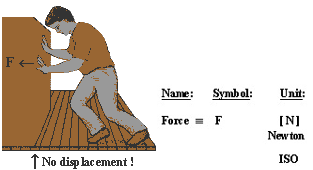Work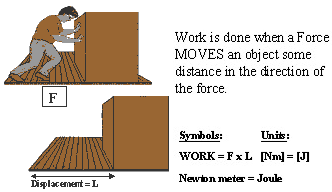#### Power

In many real-world situations not the total amount of WORK is important, but the RATE at which the work is done.
POWER is the RATE at which WORK is done.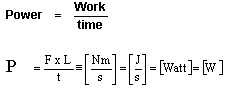Power=Work per unit of time

### Single Phase, Sine Wave Theory

#### AVERAGE POWER

Long time the power of steam and explosion engines was expressed in Horse Power (Hp, Pk).
Also the first electrical DC-current engines were compared for their maximum delivered power to their predecessor and consequently expressed in Horse Power.
In AC current driven engines, the delivered power is changing continuously every period.
You could specify such engine like :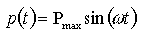In order to compare the performance of AC-engines with other engines, the term AVERAGE POWER was introduced.

#### AVERAGE-GENERAL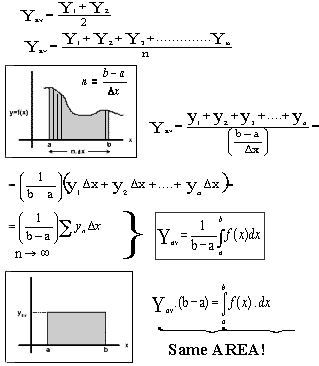#### Example Average (1)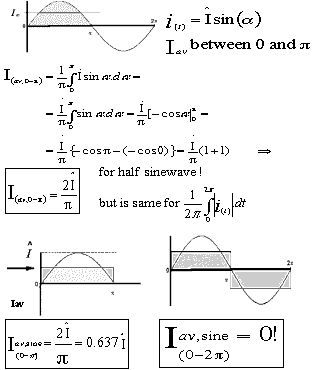#### Example Average (2)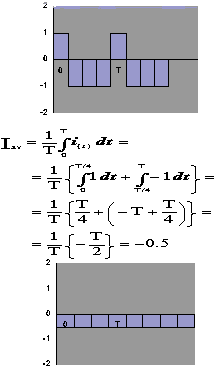#### AVERAGE CURRENT

The AVERAGE value Iav of an Alternating Current (AC) is expressed by that Direct Current (DC) which transfers across any circuit the same net charge as is transferred by that alternating current during the same time.

#### AVERAGE POWER

If power P(t) is a PERIODIC function of time t with period T, then the Average Power is: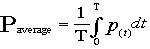This is not only true for sine waves, but for any other periodic waveform.

#### RMS-CURRENT

The RMS value of an Alternating Current (AC) is given by that Direct Current (DC) which when flowing through a given circuit for a given time, produces the same HEAT (= Energy) as produced by the alternating current when flowing through the same circuit for the same time.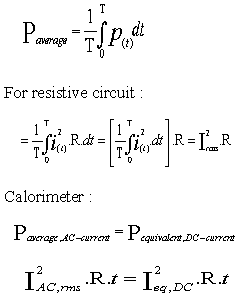#### ROOT MEAN SQUARE GENERAL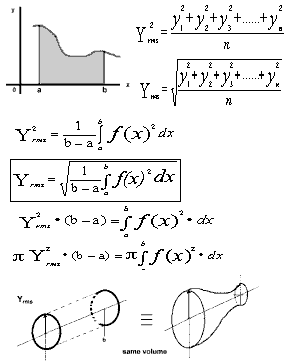#### RMS example (1): Pure Sine-wave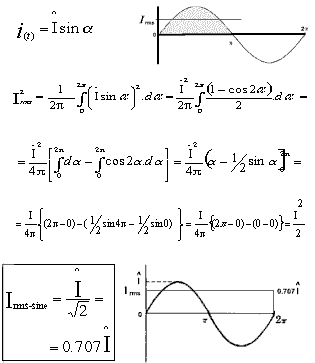#### RMS example (2) : Alternating Block-wave with 25% duty-cycle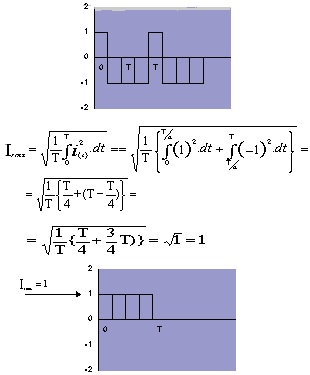#### RMS example (3): 50% Duty-Cycle Pulse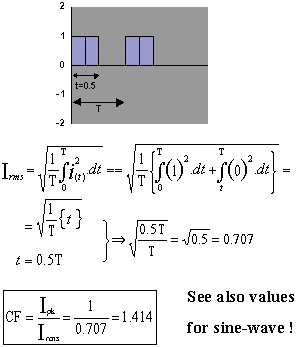#### TEST

What is Urms?
What is the Crest Factor?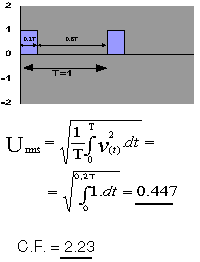### Crest Factor

#### What is Crest Factor

The crest factor is the ratio of peak value to RMS value of waveform as shown in below figure. This ratio is also called to peak-to-RMS ratio. The crest factor is expressed as follows:
Crest Factor (peak-to-RMS ratio)= (peak value)/(RMS value)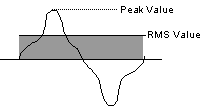For the power meter, crest factor has two meanings. One is a specification of the power meter itself. It expresses that the instrument has the capability of correct measurement how much distorted waveform. The other is the measuring of the crest factor of the input voltage or current of an input signal. It means to know quality of an input signal.

#### A measurement instrument specification

For a measuring instrument, the crest factor expresses the size of the dynamic range for an input signal. We define the crest factor as the size of dynamic range based on rated range value (RMS value).
For example, Crest factor of the WT2000 is three or six. It means possible to measure the input signal that peek value is three or six times larger than rated range value.
For example, using 100Vrms range and 1Arms range in the WT2000, available input voltage and current signals are as follows:
• The case of select CF=3 in WT2000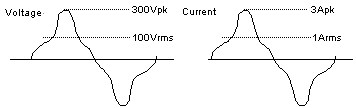• The case of select CF=6 in WT2000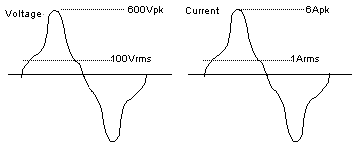A measurement instrument specification

If input RMS value is lower than rated range value, the power meter can measure signal with more large crest factor. Below table shows between available input signal and the crest factor.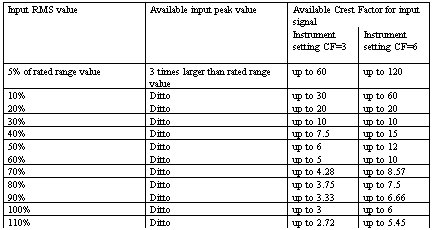Input signal range which is defined accuracy, is within 10 to 110% of rated range. Therefore, in CF=3, the crest factor as the size of dynamic range for the WT series is up to 30 (at 10% of rated range value input) within the range of defined accuracy.
For your information, the WT series can display value when it puts the signal that is more than 0.5% of rated range value. If the input RMS value is 0.5% of rated range and input peek value is three times larger than rated range value, the crest factor becomes 600.
If an input exceeding the value shown in the specifications is measured, the peak area of the waveform is clipped to cause an error.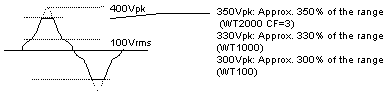#### Quality of an input signal

Below table shows RMS value, crest factor and form factor for a typical waveform. The form factor is defined as RMS value/MEAN value. The crest factor of sine wave is the square root of 2.
If it calculates crest factor of input signal and compares this crest factor with the square root of 2, it knows how much distortion ratio of input waveform.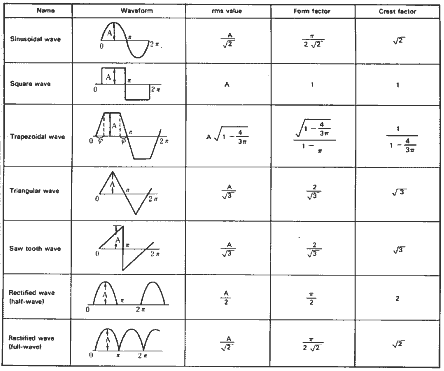Below figure shows thyristor waveform characteristic for firing angle versus crest factor.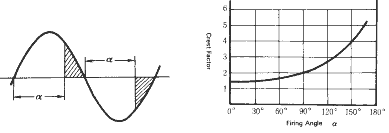Below figure shows pulse waveform characteristic of pulse duty cycle (=t/T) versus crest factor.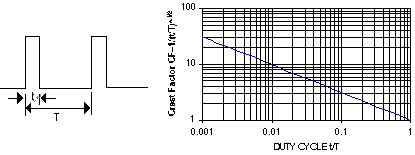### Common Mode Voltage Rejection

#### What is common mode

• The input circuitry of the power meter is floating from ground (earth potential). There is a so called stray capacitance between this input circuitry and the case.
• When a high voltage containing high frequencies is applied to the input terminals, it produces high frequency current through the input circuitry and the stray capacitance to earth.
• It causes error that the current produce the potential difference.
• Both paths not being identical causes a potential difference at the input of the DA-converter, that did originally not exist at the terminals.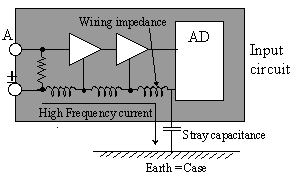Influence of common mode voltage

The measured value of a current influenced by a common mode voltage is shown below: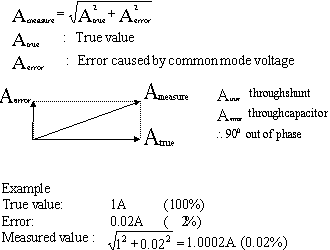NOTE: Few percentage error caused by common mode voltage can be bypassed.

#### Common mode rejection ratio specification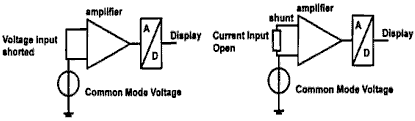An applied Common mode voltage will generate noise on the input circuitry which will result in a detected Urms and Irms on the display after A/D conversion, which is most critical for current measurements due to low shunt voltage.
YOKOGAWA specifies the effect of Common Mode Voltage as follows:
When 600Vrms at 50/60Hz Common Mode Voltage is applied to the Voltage or Current input the displayed noise value will be less then 0.01% of the selected range for all ranges. When we express the value in dB against selected range we get: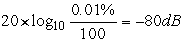Practically this means that in the 10V range the displayed value will be lower then: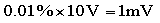This also means that in the 1A range the displayed value will be lower then: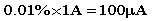The Yokogawa method gives immediately the error influence on the final result in % of the selected range. Most other manufacturers specify the Common Mode Voltage effect by using strictly the CMRR formula: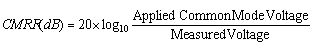For example:

• Voltage input:
120dB Common Mode Rejection Ratio at 50Hz
• Current input:
140dB Common Mode Rejection Ratio at 50Hz

When 600Vrms is applied to the inputs the maximum measured voltage can be calculated as follows: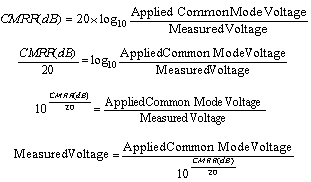For the Voltage input with 120 dB CMRR at 50Hz, the applied 600 Vrms will give a maximum measured voltage of: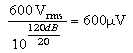In the 10V range this means a maximum error of 0.006 % of range.
For the Current input with 140 dB CMRR at 50Hz, the applied 600 Vrms will give a maximum measured voltage of:<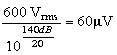In the current range the voltage should be transferred to a current value by using the formula: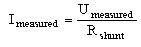When a 0.1 ohm shunt in 1 A range has been used it means that 60 mV noise is equivalent to 600 mA error which is an error of 0.06% of range.

Conclusion: Yokogawa uses a different way of specifying with the advantage that the error can easily be determined for current and voltage. Other manufacturers follow the strict CMRR formula with the disadvantage that it is rather complicated to calculate the error in the current range caused by the Common Mode Voltage.

#### The easy way to confirm the influence of common mode

The measured value for the voltage and the current should be 0 in WIRING 2.
The error in WIRING 2 can be influence by common mode voltage.
• Wiring 1.- normal wiring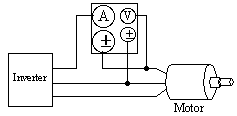• Wiring 2.- wiring to confirm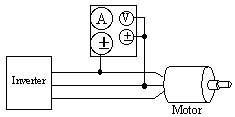#### Common Mode Voltage Rejection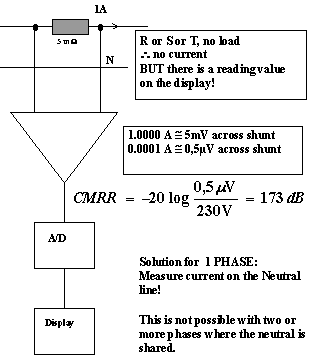### Power Factor Uncertainty

#### What is the consequence of the internal power factor uncertainty

The average electric power for a sinusoidal waveform is: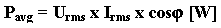Every multiplication factor contributes in its own way to the total uncertainty: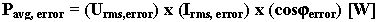When measuring power, many small errors occur. For a total inaccuracy figure we have to sum them all together. Many small possible errors result in one big uncertainty figure, so we better pay attention to all of them.

Factors that influence the total error for power are:

• Amplitude
• Frequency of the fundamental of the input signal
• Crest Factor of the input signal (BW)
• Crest Factor setting of the Power Meter
• Power Factor
• Temperature
• Use of filters

#### Effect of internal power factor at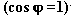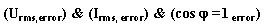When we measure voltage and current there is an uncertainty about the amplitude caused by the FREQUENCY CHARACTERISTICS (= BW=Bandwidth) of the measuring instrument itself.

Furthermore every range setting incorporates unique components, so every range will contribute differently to the total accuracy. This error is called the RANGE ERROR.

Within a range it makes a difference if the actual measurement value is close to 0% or 100% of the maximum range value. The instrument itself is calibrated at the 100% value, so this gives always the best result and close to 0% (entering the "noise floor") the worst. This error is called the READING ERROR. For Voltage, Current and Power measurements, the Error is specified as: (Error as % of reading) x reading value + (Error as % of range) x range value.

Basically this uncertainty is measured and calculated at the condition of#### Effect of internal power factor at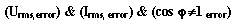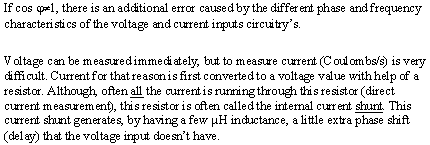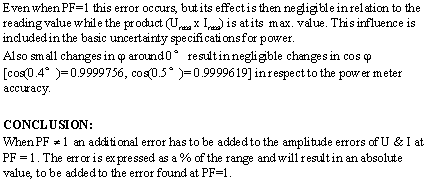#### U & I different Phase Shift

Input circuitry of the digital power meter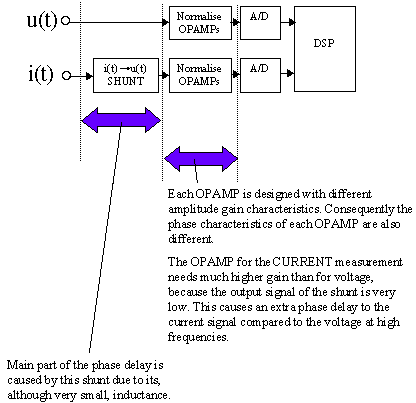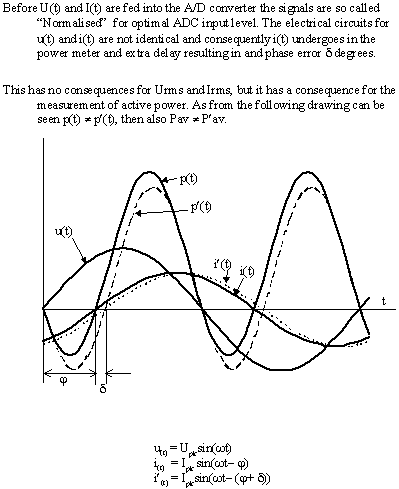An unwanted phase-shift is caused by the different phase and frequency characteristics of the voltage and current inputs circuitry's.
The current shunt generates by having a few mH inductance, a little extra phase shift to the current input. The voltage input does not have this component.
Even when PF=1 this error occurs but it's effect is neglectable at 50/60Hz in relation to the reading value while the product Urms and Irms is at its max. value.
At every frequency we have a phase delay error related to the range. This error is measured in degrees, but later specified as a % of the range for ease of use.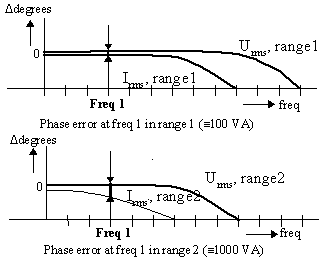If the freq. increases, the relative impact increases. At higher frequencies an extra error has to be added to the total power error. Even for the PF = 1 condition this error is no longer neglectable.

#### How to measure ε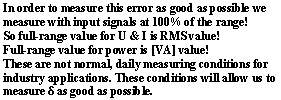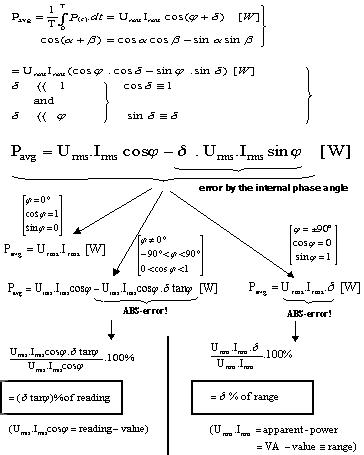#### Relation δ and Δ W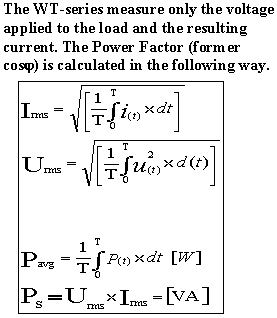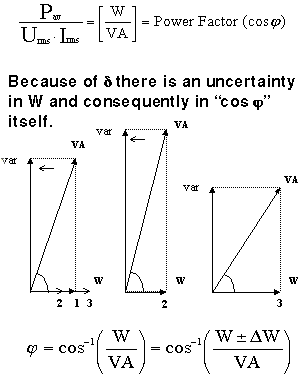### Harmonic Analysis

#### WHAT IS A HARMONIC

A harmonic is a sine wave with a multiple frequency of the fundamental wave. A distorted wave consists of a DC- component, the fundamental wave and its harmonics.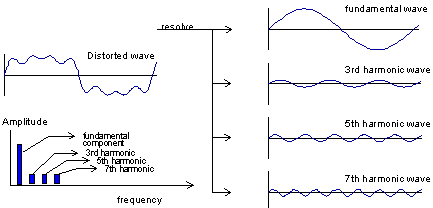Harmonic analysis is resolving a distorted wave form into the fundamental component and its harmonic components. The WT-series realises this by using FFT (except for the DC-component, which is measured in DC-mode).

#### WHY IS HARMONIC ANALYSIS NEEDED

Sometimes, non-linear loads cause harmonic current components on the mains. Because of the non-zero impedance of the power lines this results in a distorted voltage at the neighbours. Affecting badly the electric and electronics instruments connected to the same power line.

For example, it is possible that a power supply capacitor equipment produces strange sounds, vibrates and heats-up by the harmonics current. As a result, its life time decreases, it causes to burn out or it causes malfunctioning of the equipment.

Therefore the IEC developed the regulation IEC1000-3-2, in order to protect electric equipment against the harmonic current problem.
Meanwhile, inverter- and motor manufacturers use the harmonic analysis to measure the performance of their products. Now a days, many inverters are used to drive electric motors. The inverter controls the rotating speed of the motor by varying the frequency or amplitude of the input voltage to the motor. The varied signal is a distorted waveform, so it includes harmonic components. These components also are affect the motor rotation and the motor does not rotate smoothly. This means a waste of the power and shortening the motors life time. Therefore these manufacturers analyse the harmonics.

#### NFLUENCE OF WINDOW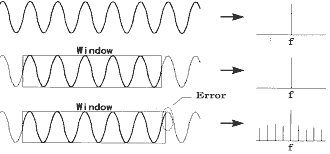#### SAMPLING SYNCHRONISATION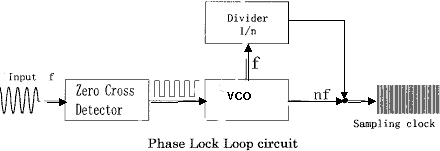• The VCO outputs a sampling clock n-times the input frequency.
• The FFT calculation is always based on n-data points

#### How many harmonics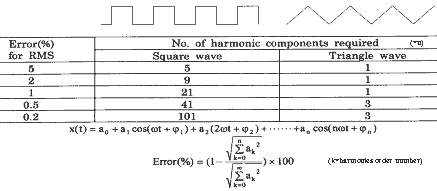This table shows the relationship between the number of harmonics included and the remaining error as a percentage of the ideal waveform.

Note

• The fundamental (= first harmonic) and the higher harmonics are pure sine-waves.
• The error is measured against RMS values

#### How many Harmonics are enough

Error when excluding higher harmonics (rectangular and triangular waves)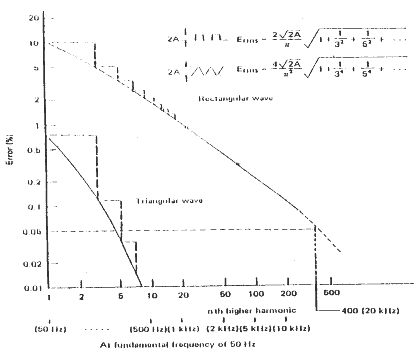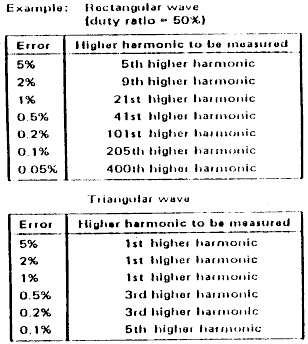### History and different types of power meters

#### Principle of Operation

1. THERMAL WATTMETER

This instrument uses a thermocouple to convert an AC-current into a DC-equivalent current (its so called RMS-value). When a current flows through the hot-wire, its temperature goes up.
A thermocouple is connected to this hot-wire, so thermocouple is also heated up.
In turn, the thermocouple generates a DC-voltage proportionally to the developed heat at the junction.
A moving coil instrument is used to indicate the result. For measuring power, The rising temperature of the thermocouple is proportional to square value of the current and equals the active power.
In this case, this instrument uses two thermocouples with a differential circuit. This instrument can be used to measure both DC and AC.
Frequency bandwidth Current: up to approx. 10 MHz
Voltage: up to approx. 100 kHz
Heat-wire is very thin, so it is easy to burn out by the influence of over current.

2. Electro-dynamic instrument

When a current flows into the fixed coil, the fixed coil generates a flux field. The mobile coil rotates in this flux field. This is the principle of this instrument. The amount of torque to rotate of the mobile coil is proportional to multiplication of the current flowing through the fixed coil and the mobile coil.
To flow the difference current into the fixed coil and the mobile coil, this instrument indicates averaging value which is integrated multiplication value of the each instantaneous current by time. For measuring power, it use this characteristic.
Measuring voltage to attach resistance to the mobile coil and measuring current to flow current into the fixed coil, this instrument indicates multiplication value between voltage and current. It is power.
This instrument can use to measure DC and AC.
Frequency bandwidth current: up to approx. 1 kHz
voltage: up to approx. 150 Hz
*YOKOGAWA 2041,2042 ( DC, 25 Hz to 1000 Hz)

3. Induction instrument

Putting a conductor into a moving or rotating flux field generates a so called eddy current in the conductor. The force between this eddy current and the flux field, moves or rotates the conductor. This instrument uses this driving force.

#### Operating Principle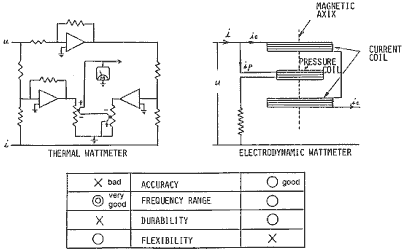#### Measurement Principle of a Wattmeter

An electrodynamics wattmeter is composed of a pair of fixed coils and one mobile coil. The mobile coil moves in a spinning direction in the magnetic field generated by the fixed coils. The torque applied to the mobile coil is proportional to the product of the currents flowing in the fixed and mobile coils. When a load current flows in the fixed coils and a small current proportional to the load current flows in the mobile coil, the torque on the mobile coil is proportional to the product of the load current and voltage. For an alternating current, the phase-angles of the currents in both coils
(i.e., phase angles of the currents against the voltages) affects the torque in the same relationship as the power of the load power. This means that the torque is proportional to the electric power whether the current is alternating or direct, and the power can be indicated by this meter mechanism.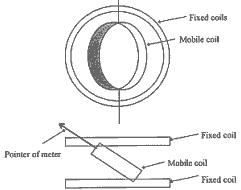#### Semi-Digital Type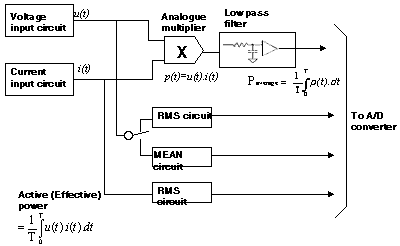#### Analog Computation Wattmeter

An analog computation wattmeter computes the instantaneous electric power with the full use of an analog switching circuit. It generates a constant-period pulse-width modulation waveform proportional to the input level of the voltage or current (such that the duty of each cycle of the pulse is proportional to the input level), and superimposes the input current (or voltage) waveform on that pulse waveform to obtain a multiplication of the voltage and current waveforms. This method is referred to as time-division multiplication. At the last stage, the effective power value is obtained from the time-division multiplication waveform through averaging by an analog filter circuit.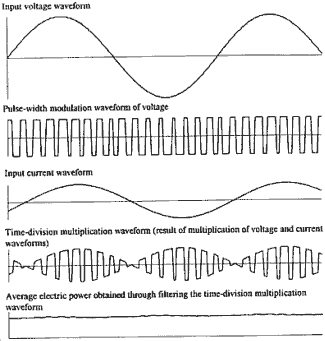#### Digital Type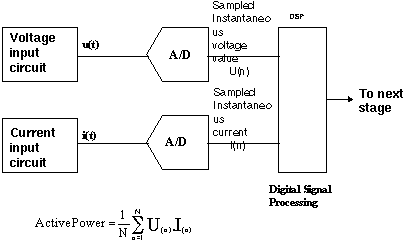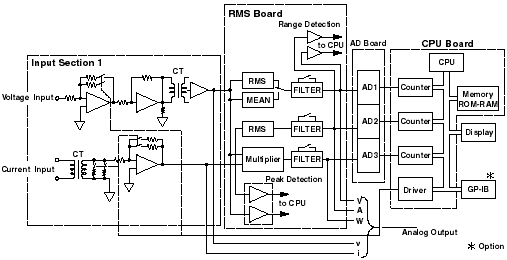#### Measurement Principle of a Digital Sampling Power Meter

In general, the following equation is used to express the effective power, where the instantaneous power values, products of the instantaneous voltage and current values, are integrated and averaged by cycle T.
• u(t): instantaneous voltage value at time t
• i (t) instantaneous current value at time t
• u (k): instantaneous voltage value at k-th sample
• i (k) instantaneous current value at k-th sample
• T : cycle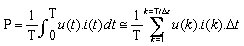From the approximation on the right above, we can tell that the effective power can be obtained by averaging the number of k (=T/ _ t) segments of the width of _ t by cycle T. A digital sampling wattmeter executes this computation almost as is. In an actual wattmeter, the waveform measurement time period is often set longer than one cycle. _ t is generally around tens of microseconds and the sampling frequency is the inverse number of the _ t.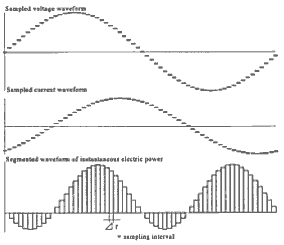Click here for an example of a Full-Digital Type: Yokogawa WT 2030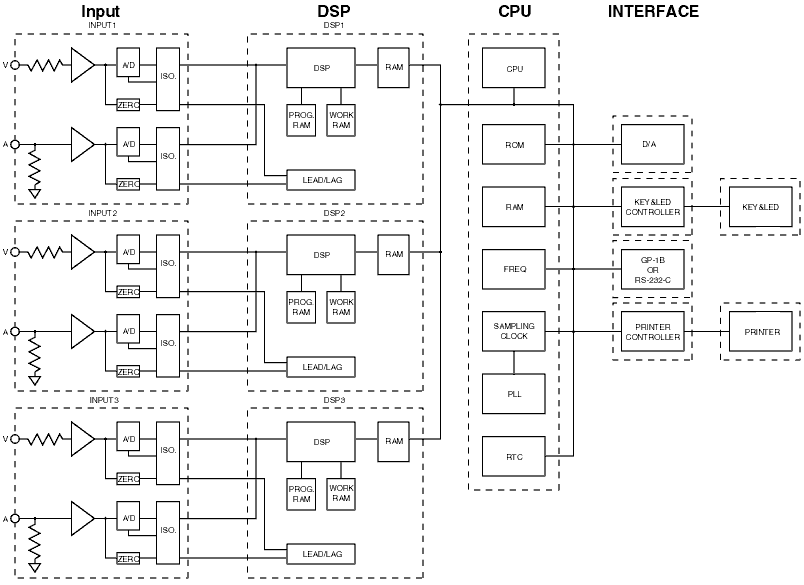#### Measurement Functions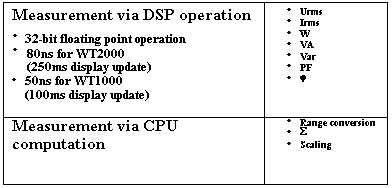### Related Products & Solutions

#### WT2010/WT2030 Digital Power Meters

The WT2000 digital powermeter series has been designed with emphasis on basic performance (bandwidth, accuracy, response speed, and noise immunity) from the viewpoint of measurement of electrical quantities. The broad range of functions of these power analyzers enable them to be used in various fields of applications.

Top